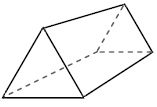# Vertices, Edges, and Faces of a Solid Online Quiz

Following quiz provides Multiple Choice Questions (MCQs) related to Vertices, Edges, and Faces of a Solid. You will have to read all the given answers and click over the correct answer. If you are not sure about the answer then you can check the answer using Show Answer button. You can use Next Quiz button to check new set of questions in the quiz.Q 1 - Determine the faces, vertices and edges of the following shape.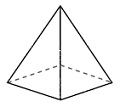### Explanation

Step 1:

The given shape is a square pyramid.

Step 2:

It has 5 faces, 5 vertices, and 8 edges.

Q 2 - Determine the faces, vertices and edges of the following shape.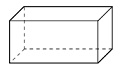### Explanation

Step 1:

The given shape is a rectangular prism.

Step 2:

It has 6 faces, 8 vertices, and 12 edges.

Q 3 - Determine the faces, vertices and edges of the following shape.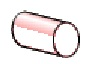### Explanation

Step 1:

The given shape is a cylinder.

Step 2:

It has 2 faces, 0 vertices, and 0 edges.

Q 4 - Determine the faces, vertices and edges of the following shape.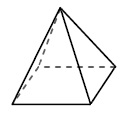### Explanation

Step 1:

The given shape is a square pyramid.

Step 2:

It has 5 faces, 5 vertices, and 8 edges.

Q 5 - Determine the faces, vertices and edges of the following shape.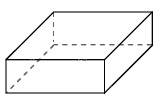### Explanation

Step 1:

The given shape is a rectangular prism.

Step 2:

It has 6 faces, 8 vertices, and 12 edges.

Q 6 - Determine the faces, vertices and edges of the following shape.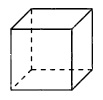### Explanation

Step 1:

The given shape is a cube.

Step 2:

It has 6 faces, 8 vertices, and 12 edges.

Q 7 - Determine the faces, vertices and edges of the following shape.### Explanation

Step 1:

The given shape is a sphere.

Step 2:

It has 0 faces, 0 vertices, and 0 edges.

Q 8 - Determine the faces, vertices and edges of the following shape.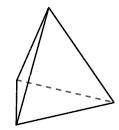### Explanation

Step 1:

The given shape is a triangular pyramid.

Step 2:

It has 4 faces, 4 vertices, and 6 edges.

Q 9 - Determine the faces, vertices and edges of the following shape.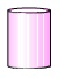### Explanation

Step 1:

The given shape is a cylinder.

Step 2:

It has 2 faces, 0 vertices, and 0 edges.

Q 10 - Determine the faces, vertices and edges of the following shape.